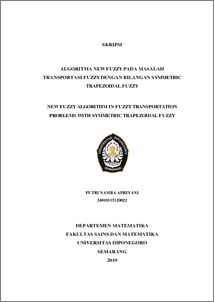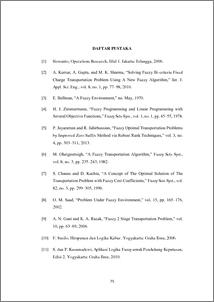# NEW FUZZY ALGORITHM IN FUZZY TRANSPORTATION PROBLEMS WITH SYMMETRIC TRAPEZOIDAL FUZZY

Apriyani, Putri Namba (2019) NEW FUZZY ALGORITHM IN FUZZY TRANSPORTATION PROBLEMS WITH SYMMETRIC TRAPEZOIDAL FUZZY. Undergraduate thesis, UNDIP.Preview
PDF
99KbPDF
Restricted to Repository staff only

1762KbPreview
PDF
108Kb

## Abstract

The problem of transportation with fixed charges is one form of fuzzy transportation problems where the parameters transportation costs and fixed charges are in the form of fuzzy numbers. In fuzzy transportation problems with fixed charges, fuzzy numbers used are trapezoidal fuzzy symmetric numbers. The solution to fuzzy transportation problems with fixed charges using trapezoidal fuzzy symmetric numbers is using the New Fuzzy Algorithm. The New fuzzy algorithm provides the optimal solution that has been shown. In this Thesis, the results of the New Fuzzy Algorithm provide an optimal solution to fuzzy transportation problems. Keyword : Fuzzy Transportation Problems, Fixed Charge Transportation Problems, New Fuzzy Algorithm, Symmetric Trapezoidal Fuzzy Numbers

Item Type: Thesis (Undergraduate) Q Science > QA Mathematics Faculty of Science and Mathematics > Department of Mathematics 84214 INVALID USER 11 Jun 2022 15:08 11 Jun 2022 15:08

Repository Staff Only: item control page